NEET  >  31 Year NEET Previous Year Questions: Laws of Motion - 2

# 31 Year NEET Previous Year Questions: Laws of Motion - 2

Test Description

## 30 Questions MCQ Test Physics Class 11 | 31 Year NEET Previous Year Questions: Laws of Motion - 2

31 Year NEET Previous Year Questions: Laws of Motion - 2 for NEET 2023 is part of Physics Class 11 preparation. The 31 Year NEET Previous Year Questions: Laws of Motion - 2 questions and answers have been prepared according to the NEET exam syllabus.The 31 Year NEET Previous Year Questions: Laws of Motion - 2 MCQs are made for NEET 2023 Exam. Find important definitions, questions, notes, meanings, examples, exercises, MCQs and online tests for 31 Year NEET Previous Year Questions: Laws of Motion - 2 below.
Solutions of 31 Year NEET Previous Year Questions: Laws of Motion - 2 questions in English are available as part of our Physics Class 11 for NEET & 31 Year NEET Previous Year Questions: Laws of Motion - 2 solutions in Hindi for Physics Class 11 course. Download more important topics, notes, lectures and mock test series for NEET Exam by signing up for free. Attempt 31 Year NEET Previous Year Questions: Laws of Motion - 2 | 30 questions in 60 minutes | Mock test for NEET preparation | Free important questions MCQ to study Physics Class 11 for NEET Exam | Download free PDF with solutions
 1 Crore+ students have signed up on EduRev. Have you?
31 Year NEET Previous Year Questions: Laws of Motion - 2 - Question 1

### Starting from rest, a body slides down a 45° inclined plane in twice the time it takes to slide down the same distance in the absence of friction.The coefficient of friction between the body and the inclined plane is 

Detailed Solution for 31 Year NEET Previous Year Questions: Laws of Motion - 2 - Question 1

In presence of friction a = (g sinθ – μg cosθ)

∴   Time taken to slide down the plane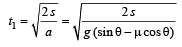In absence of friction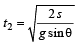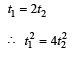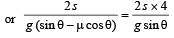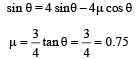31 Year NEET Previous Year Questions: Laws of Motion - 2 - Question 2

### Two bodies of masses m and 4m are moving with equal kinetic energies. The ratio of their linear momenta will be 

Detailed Solution for 31 Year NEET Previous Year Questions: Laws of Motion - 2 - Question 2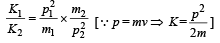Hence,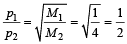31 Year NEET Previous Year Questions: Laws of Motion - 2 - Question 3

### A body of mass 5 kg explodes at rest into three fragments with masses in the ratio 1 : 1 : 3. The fragments with equal masses fly in mutually perpendicular directions with speeds of 21 m/s.The velocity of heaviest fragment in m/s will be

Detailed Solution for 31 Year NEET Previous Year Questions: Laws of Motion - 2 - Question 3

Masses of the pieces are 1, 1, 3 kg. Hence

(1 x 21)2 + (1 x 21)2 = (3 x V)2

That is,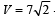31 Year NEET Previous Year Questions: Laws of Motion - 2 - Question 4

A 4 kg mass and 1 kg are moving with equal kinetic energies. The ratio of the magnitudes of their linear momenta is 

Detailed Solution for 31 Year NEET Previous Year Questions: Laws of Motion - 2 - Question 4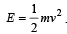. Hence, mv = (2mE)1/2. For same

KE, momentum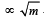Hence, the ratio is 2 : 1.

31 Year NEET Previous Year Questions: Laws of Motion - 2 - Question 5

A 600 kg rocket is set for a vertical firing. If the exhaust speed is 1000 ms–1 , the mass of the gas ejected per second to supply the thrust needed to overcome the weight of rocket is 

Detailed Solution for 31 Year NEET Previous Year Questions: Laws of Motion - 2 - Question 5

Thrust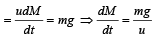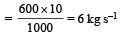31 Year NEET Previous Year Questions: Laws of Motion - 2 - Question 6

A particle of mass m is moving with a uniform velocity v1. It is given an impulse such that its velocity becomes v2. The impulse is equal to 

Detailed Solution for 31 Year NEET Previous Year Questions: Laws of Motion - 2 - Question 6

Impulse = final momentum – initial momentum = m (v2 – v1)

31 Year NEET Previous Year Questions: Laws of Motion - 2 - Question 7

A heavy uniform chain lies on horizontal table top. If the coefficient of friction between the chain and the table surface is 0.25, then the maximum fraction of the length of the chain that can hang over one edge of the table is 

Detailed Solution for 31 Year NEET Previous Year Questions: Laws of Motion - 2 - Question 7

The force of friction on the chain lying on the table should be equal to the weight of the hanging chain.
Let ρ = mass per unit length of the chain
µ = coefficient of friction
l = length of the total chain
x = length of hanging chain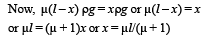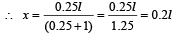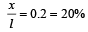31 Year NEET Previous Year Questions: Laws of Motion - 2 - Question 8

Physical independence of force is a consequence of

Detailed Solution for 31 Year NEET Previous Year Questions: Laws of Motion - 2 - Question 8

Newton’s first law of motion is related to physical independence of force.

31 Year NEET Previous Year Questions: Laws of Motion - 2 - Question 9

Consider a car moving along a straight horizontal road  with a speed of 72 km/h. If the coefficient of static friction between the tyres and the road is 0.5, the shortest distance in which the car can be stopped is (taking g = 10 m/s2) 

Detailed Solution for 31 Year NEET Previous Year Questions: Laws of Motion - 2 - Question 9

Here u = 72 km/h = 20 m/s; v = 0;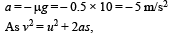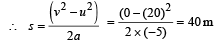31 Year NEET Previous Year Questions: Laws of Motion - 2 - Question 10

A particle of mass M is moving in a horizontal circle of radius R with uniform speed V. When it moves from one point to a diametrically opposite point, its 

Detailed Solution for 31 Year NEET Previous Year Questions: Laws of Motion - 2 - Question 10

On the diametrically opposite points, the velocities have same magnitude but opposite directions. Therefore, change in momentum is MV – (– MV) = 2MV

31 Year NEET Previous Year Questions: Laws of Motion - 2 - Question 11

A monkey is decending from the branch of a tree with constant acceleration. If the breaking strength is 75% of the weight of the monkey, the minimum acceleration with which monkey can slide down without breaking the branch is 

Detailed Solution for 31 Year NEET Previous Year Questions: Laws of Motion - 2 - Question 11

Let T be the tension in the branch of a tree when monkey is descending with acceleration a. Then mg – T = ma; and T = 75% of weight of monkey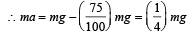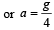31 Year NEET Previous Year Questions: Laws of Motion - 2 - Question 12

A block has been placed on an inclined plane with the slope angle θ, block slides down the plane at constant speed. The coefficient of kinetic friction is equal to 

Detailed Solution for 31 Year NEET Previous Year Questions: Laws of Motion - 2 - Question 12

When the block slides down the plane with a constant speed, then the inclination of the plane is equal to angle of repose (θ).
Coeff. of friction = tan of the angle of repose = tanθ.

31 Year NEET Previous Year Questions: Laws of Motion - 2 - Question 13

A shell is fired from a cannon, it explodes in mid air, its total 

Detailed Solution for 31 Year NEET Previous Year Questions: Laws of Motion - 2 - Question 13

When shell explodes in mid air its chemical energy is partly converted into mechanical energy, hence K.E. increases.

31 Year NEET Previous Year Questions: Laws of Motion - 2 - Question 14

A satellite in a force free space sweeps stationary interplanetary dust at a rate (dM/dt) = αv. The acceleration of satellite is 

Detailed Solution for 31 Year NEET Previous Year Questions: Laws of Motion - 2 - Question 14

Thrust on the satellite,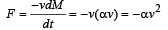Acceleration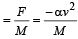31 Year NEET Previous Year Questions: Laws of Motion - 2 - Question 15

If the force on a rocket moving with a velocity of 300 m/sec is 345 N, then the rate of combustion of the fuel, is 

Detailed Solution for 31 Year NEET Previous Year Questions: Laws of Motion - 2 - Question 15

Velocity of the rocket (u) = 300 m/s and force (F) = 345N. Rate of combustion of fuel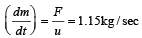31 Year NEET Previous Year Questions: Laws of Motion - 2 - Question 16

What will be the maximum speed of a car on a road turn of radius 30 m if the coefficient of friction between the tyres and the road is 0.4 (Take g = 9.8 m/s2) 

Detailed Solution for 31 Year NEET Previous Year Questions: Laws of Motion - 2 - Question 16

r = 30 m and μ = 0.4.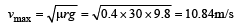31 Year NEET Previous Year Questions: Laws of Motion - 2 - Question 17

A 10 N force is applied on a body produces an acceleration of 1 m/s2. The mass of the body is 

Detailed Solution for 31 Year NEET Previous Year Questions: Laws of Motion - 2 - Question 17

By Newton's IInd law of motion,  F = ma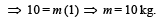31 Year NEET Previous Year Questions: Laws of Motion - 2 - Question 18

A ball of mass 150 g, moving with an acceleration 20 m/s2, is hit by a force, which acts on it for 0.1 sec. The impulsive force is 

Detailed Solution for 31 Year NEET Previous Year Questions: Laws of Motion - 2 - Question 18

Mass = 150 gm =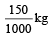Force = Mass × acceleration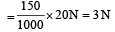Impulsive force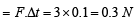31 Year NEET Previous Year Questions: Laws of Motion - 2 - Question 19

A mass of 1 kg is suspended by a thread. It is (i) lifted up with an acceleration 4.9 m/s2, (ii) lowered with an acceleration 4.9 m/s2.The ratio of the tensions is 

Detailed Solution for 31 Year NEET Previous Year Questions: Laws of Motion - 2 - Question 19

In case (i) we have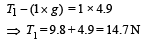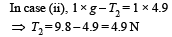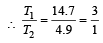31 Year NEET Previous Year Questions: Laws of Motion - 2 - Question 20

A bullet is fired from a gun. The force on the bullet is given by F = 600 – 2 × 105 t where, F is in newton and t in second. The force on the bullet becomes zero as soon as it leaves the barrel.What is the average impulse imparted to the bullet?

Detailed Solution for 31 Year NEET Previous Year Questions: Laws of Motion - 2 - Question 20

Given F = 600 – (2 x 105t ) The force is zero at time t, given by
0 = 600 – 2 x 105t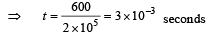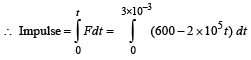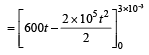= 600 x 3 x 10 –3 – 105 (3 x10 –3)2
= 1.8 – 0.9 = 0.9Ns

31 Year NEET Previous Year Questions: Laws of Motion - 2 - Question 21

A 5000 kg rocket is set for vertical firing. The exhaust speed is 800 ms–1. To give an initial upward acceleration of 20 ms–2, the amount of gas ejected per second to supply the needed thrust will be (g = 10 ms–2) 

Detailed Solution for 31 Year NEET Previous Year Questions: Laws of Motion - 2 - Question 21

Given : Mass of rocket (m) = 5000 kg
Exhaust speed (v) = 800 m/s
Acceleration of rocket (a) = 20 m/s2
Gravitational acceleration (g) = 10 m/s2
We know that upward force F = m (g + a) = 5000 (10 +20)
= 5000 × 30 = 150000 N.
We also know that amount of gas ejected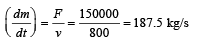31 Year NEET Previous Year Questions: Laws of Motion - 2 - Question 22

Two blocks m1 = 5 gm and m2 = 10 gm are hung vertically over a light frictionless pulley as shown here. What is the acceleration of the masses when they are left free?        (where g is acceleration due to gravity)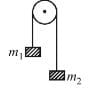Detailed Solution for 31 Year NEET Previous Year Questions: Laws of Motion - 2 - Question 22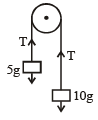Let T be the tension in the string.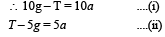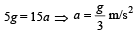31 Year NEET Previous Year Questions: Laws of Motion - 2 - Question 23

A person slides freely down a frictionless inclined plane while his bag falls down vertically from the same height. The final speeds of the man (VM) and the bag (VB) should be such that 

Detailed Solution for 31 Year NEET Previous Year Questions: Laws of Motion - 2 - Question 23

As there is only gravitational field which works.
We know it is conservative field and depends only on the end points.  So, VM = VB

31 Year NEET Previous Year Questions: Laws of Motion - 2 - Question 24

A block of mass 1 kg lies on a horizontal surface in a truck the coefficient of static friction between the block and the surface is 0.6. If the acceleration of the truck is 5 m/s2, the frictional force acting on the block is

Detailed Solution for 31 Year NEET Previous Year Questions: Laws of Motion - 2 - Question 24

The maximum frictional force acting will be μmg = 6N

But pseudo force acting on the body is ma = 1×5 = 5N

Since pseudo force < frictional force

the frictional force acting is only 5N

31 Year NEET Previous Year Questions: Laws of Motion - 2 - Question 25

If a cricketer catches a ball of mass 150 gm moving with a velocity of 20 m/s, then he experiences a force of (Time taken to complete the catch is 0.1 sec.)

Detailed Solution for 31 Year NEET Previous Year Questions: Laws of Motion - 2 - Question 25

Net force experienced =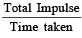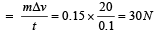31 Year NEET Previous Year Questions: Laws of Motion - 2 - Question 26

A lift weighing 1000 kg is moving upwards with an accelertion of  1 m/s2. The tension in the supporting cable is 

Detailed Solution for 31 Year NEET Previous Year Questions: Laws of Motion - 2 - Question 26

T – (1000 × 9.8) = 1000 × 1

⇒  T = 10800N

31 Year NEET Previous Year Questions: Laws of Motion - 2 - Question 27

A 100 N force acts horizontally on a block of 10 kg placed on a horizontal rough surface of coefficient of friction  μ = 0.5. If the acceleration due to gravity (g) is taken as 10 ms–2, the acceleration of the block (in ms–2) is 

Detailed Solution for 31 Year NEET Previous Year Questions: Laws of Motion - 2 - Question 27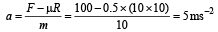31 Year NEET Previous Year Questions: Laws of Motion - 2 - Question 28

A man weighing 80 kg, stands on a weighing scale in a lift which is moving upwards with a uniform acceleration of 5m/s2. What would be the reading on the scale ? (g = 10 m/s2) 

Detailed Solution for 31 Year NEET Previous Year Questions: Laws of Motion - 2 - Question 28

Reading of the scale = Apparent wt. of the man = m(g + a) = 80 (10 + 5) = 1200 N

31 Year NEET Previous Year Questions: Laws of Motion - 2 - Question 29

A monkey of mass 20 kg is holding a vertical rope. The rope will not break when a mass of 25 kg is suspended from it but will break if the mass exceeds 25 kg. What is the maximum acceleration with which the monkey can climb up along the rope ? (g = 10 m/s2) 

Detailed Solution for 31 Year NEET Previous Year Questions: Laws of Motion - 2 - Question 29

T = Tension caused in string by monkey
= m (g + a)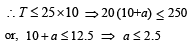31 Year NEET Previous Year Questions: Laws of Motion - 2 - Question 30

The coeffici ent of static friction , μs, between block A of mass 2 kg and the table as shown in the figure is 0.2. What would be the maximum mass value of block B so that the two blocks do not move? The string and the pulley are assumed to be smooth and massless. (g = 10 m/s2)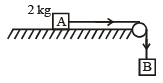Detailed Solution for 31 Year NEET Previous Year Questions: Laws of Motion - 2 - Question 30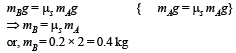## Physics Class 11

127 videos|464 docs|210 tests
 Use Code STAYHOME200 and get INR 200 additional OFF Use Coupon Code
Information about 31 Year NEET Previous Year Questions: Laws of Motion - 2 Page
In this test you can find the Exam questions for 31 Year NEET Previous Year Questions: Laws of Motion - 2 solved & explained in the simplest way possible. Besides giving Questions and answers for 31 Year NEET Previous Year Questions: Laws of Motion - 2, EduRev gives you an ample number of Online tests for practice

## Physics Class 11

127 videos|464 docs|210 tests

### How to Prepare for NEET

Read our guide to prepare for NEET which is created by Toppers & the best Teachers